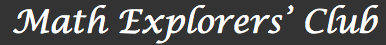Introduction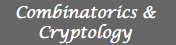Combinatorics Cryptography Number Theory and  Cryptography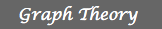Graph theory I Graph theory II Mathematics of Web Search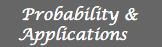Auctions Benford's Law Elementary Probability and  Applications Game Theory Markov Chains Mathematical Finance Probability Games Random Number GeneratorsCardinality, Constructibility and  Paradoxes Cardinality of sets Ehrenfeucht-Fraïssé games Gödel's Incompleteness  Theorem Puzzles and Paradoxes in  Mathematic Induction Puzzles and Paradoxes: Infinity in  Finite Terms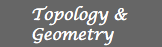Billiards and Puzzles Chaos and Fractals Escher and Hyperbolic  Geometry Geometric Dissections Geometry of Minimal Surfaces Groups and Geometries Groups of symmetries Knot Theory Mathematics of Distance Non-Euclidean Geometry The Geometry of Flat Objects in  Many Dimensions Tilings Topology and Geometry of  Surfaces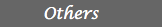Error Correcting Codes Fast Computations Games I Games II KenKen Puzzles Linkages, Configurations and  Complex Numbers Mathematics and Sudoku Puzzles Puzzles II The Math behind Sudoku Voting and ElectionsIntroduction

The Math Explorers' Club is an NSF supported project that develops materials and activites to give middle school and high school students an experience of more advanced topics in mathematics. These materials are directed towards teachers, who are welcome to adapt and copy the materials in any way they see fit, as well as towards more advanced students, who may wish to learn independently.

The Math Explorers' Club materials come in independent self-contained sections, called modules. Each module teaches material associated with a particular theme and is aimed at students with a particular level of mathematical sophistication. These modules can be catogorized into some major branches of mathematics. The following modules have been developed for each branch: